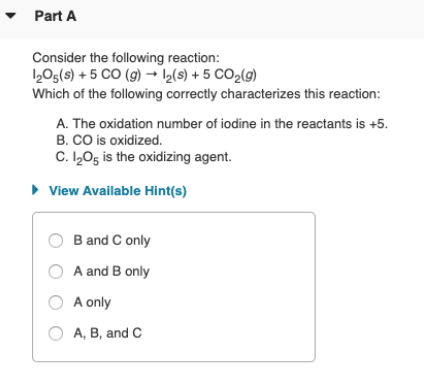# Consider the following reaction: I2O5(s) + 5CO (g) → I2(s) + 5 CO2(g) Which of the following correctly characterizes this reaction: A. The oxidation number of iodine in the reactants is +5. B. CO is oxidized. C. I2O5 is the oxidizing agent. a) B and C only b) A and B only c) A only d) A, B, and C